Shortcuts

# 第三章：PyTorch 转 ONNX 详解¶

ONNX 是目前模型部署中最重要的中间表示之一。学懂了 ONNX 的技术细节，就能规避大量的模型部署问题。从这篇文章开始，在接下来的三篇文章里，我们将由浅入深地介绍 ONNX 相关的知识。在第一篇文章里，我们会介绍更多 PyTorch 转 ONNX 的细节，让大家完全掌握把简单的 PyTorch 模型转成 ONNX 模型的方法；在第二篇文章里，我们将介绍如何在 PyTorch 中支持更多的 ONNX 算子，让大家能彻底走通 PyTorch 到 ONNX 这条部署路线；第三篇文章里，我们讲介绍 ONNX 本身的知识，以及修改、调试 ONNX 模型的常用方法，使大家能自行解决大部分和 ONNX 有关的部署问题。

## torch.onnx.export 细解¶

### 计算图导出方法¶

TorchScript 是一种序列化和优化 PyTorch 模型的格式，在优化过程中，一个torch.nn.Module模型会被转换成 TorchScript 的torch.jit.ScriptModule模型。现在， TorchScript 也被常当成一种中间表示使用。我们在其他文章中对 TorchScript 有详细的介绍，这里介绍 TorchScript 仅用于说明 PyTorch 模型转 ONNX的原理。 torch.onnx.export中需要的模型实际上是一个torch.jit.ScriptModule。而要把普通 PyTorch 模型转一个这样的 TorchScript 模型，有跟踪（trace）和脚本化（script）两种导出计算图的方法。如果给torch.onnx.export传入了一个普通 PyTorch 模型（torch.nn.Module)，那么这个模型会默认使用跟踪的方法导出。这一过程如下图所示：import torch

class Model(torch.nn.Module):
def __init__(self, n):
super().__init__()
self.n = n
self.conv = torch.nn.Conv2d(3, 3, 3)

def forward(self, x):
for i in range(self.n):
x = self.conv(x)
return x

models = [Model(2), Model(3)]
model_names = ['model_2', 'model_3']

for model, model_name in zip(models, model_names):
dummy_input = torch.rand(1, 3, 10, 10)
dummy_output = model(dummy_input)
model_trace = torch.jit.trace(model, dummy_input)
model_script = torch.jit.script(model)

# 跟踪法与直接 torch.onnx.export(model, ...)等价
torch.onnx.export(model_trace, dummy_input, f'{model_name}_trace.onnx', example_outputs=dummy_output)
# 脚本化必须先调用 torch.jit.sciprt
torch.onnx.export(model_script, dummy_input, f'{model_name}_script.onnx', example_outputs=dummy_output)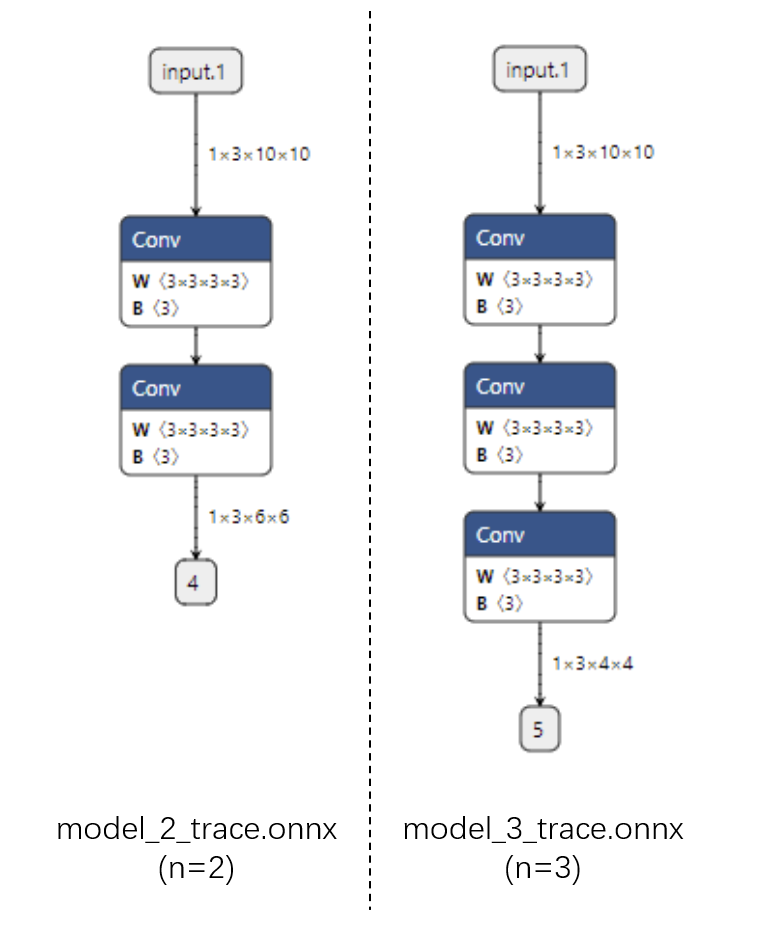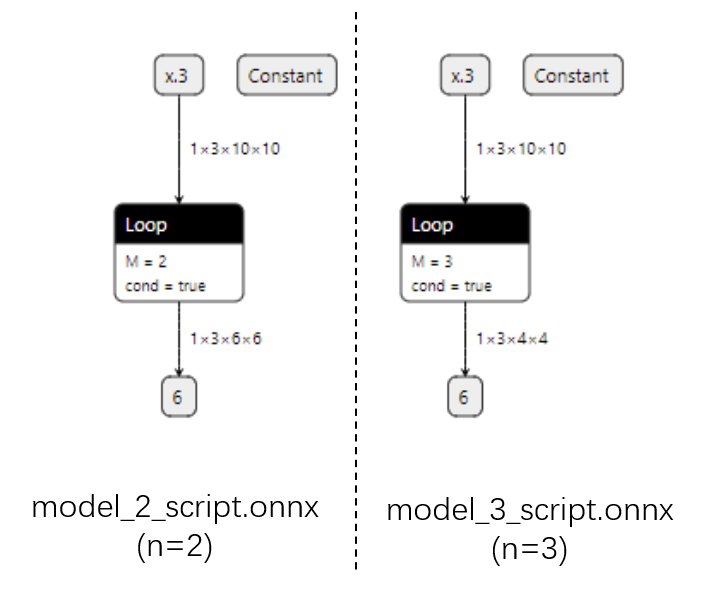### 参数讲解¶

torch.onnx.exporttorch.onnx.__init__.py文件中的定义如下：

def export(model, args, f, export_params=True, verbose=False, training=TrainingMode.EVAL,
input_names=None, output_names=None, aten=False, export_raw_ir=False,
operator_export_type=None, opset_version=None, _retain_param_name=True,
do_constant_folding=True, example_outputs=None, strip_doc_string=True,
dynamic_axes=None, keep_initializers_as_inputs=None, custom_opsets=None,
enable_onnx_checker=True, use_external_data_format=False):


#### dynamic_axes¶

import torch

class Model(torch.nn.Module):
def __init__(self):
super().__init__()
self.conv = torch.nn.Conv2d(3, 3, 3)

def forward(self, x):
x = self.conv(x)
return x

model = Model()
dummy_input = torch.rand(1, 3, 10, 10)
model_names = ['model_static.onnx',
'model_dynamic_0.onnx',
'model_dynamic_23.onnx']

dynamic_axes_0 = {
'in' : ,
'out' : 
}
dynamic_axes_23 = {
'in' : [2, 3],
'out' : [2, 3]
}

torch.onnx.export(model, dummy_input, model_names,
input_names=['in'], output_names=['out'])
torch.onnx.export(model, dummy_input, model_names,
input_names=['in'], output_names=['out'], dynamic_axes=dynamic_axes_0)
torch.onnx.export(model, dummy_input, model_names,
input_names=['in'], output_names=['out'], dynamic_axes=dynamic_axes_23)


dynamic_axes_0 = {
'in' : ,
'out' : 
}


python
dynamic_axes_0 = {
'in' : {0: 'batch'},
'out' : {0: 'batch'}
}


import onnxruntime
import numpy as np

origin_tensor = np.random.rand(1, 3, 10, 10).astype(np.float32)
mult_batch_tensor = np.random.rand(2, 3, 10, 10).astype(np.float32)
big_tensor = np.random.rand(1, 3, 20, 20).astype(np.float32)

inputs = [origin_tensor, mult_batch_tensor, big_tensor]
exceptions = dict()

for model_name in model_names:
for i, input in enumerate(inputs):
try:
ort_session = onnxruntime.InferenceSession(model_name)
ort_inputs = {'in': input}
ort_session.run(['out'], ort_inputs)
except Exception as e:
exceptions[(i, model_name)] = e
print(f'Input[{i}] on model {model_name} error.')
else:
print(f'Input[{i}] on model {model_name} succeed.')


Input on model model_static.onnx succeed.
Input on model model_static.onnx error.
Input on model model_static.onnx error.
Input on model model_dynamic_0.onnx succeed.
Input on model model_dynamic_0.onnx succeed.
Input on model model_dynamic_0.onnx error.
Input on model model_dynamic_23.onnx succeed.
Input on model model_dynamic_23.onnx error.
Input on model model_dynamic_23.onnx succeed.


print(exceptions[(1, 'model_static.onnx')])

# output
# [ONNXRuntimeError] : 2 : INVALID_ARGUMENT : Got invalid dimensions for input: in for the following indices index: 0 Got: 2 Expected: 1 Please fix either the inputs or the model.


### 使用技巧¶

#### 使模型在 ONNX 转换时有不同的行为¶

import torch

class Model(torch.nn.Module):
def __init__(self):
super().__init__()
self.conv = torch.nn.Conv2d(3, 3, 3)

def forward(self, x):
x = self.conv(x)
if torch.onnx.is_in_onnx_export():
x = torch.clip(x, 0, 1)
return x


#### 利用中断张量跟踪的操作¶

PyTorch 转 ONNX 的跟踪导出法是不是万能的。如果我们在模型中做了一些很“出格”的操作，跟踪法会把某些取决于输入的中间结果变成常量，从而使导出的ONNX模型和原来的模型有出入。以下是一个会造成这种“跟踪中断”的例子：

class Model(torch.nn.Module):
def __init__(self):
super().__init__()

def forward(self, x):
x = x * x.item()
return x, torch.Tensor([i for i in x])

model = Model()
dummy_input = torch.rand(10)
torch.onnx.export(model, dummy_input, 'a.onnx')


## PyTorch 对 ONNX 的算子支持¶

• 该算子可以一对一地翻译成一个 ONNX 算子。

• 该算子在 ONNX 中没有直接对应的算子，会翻译成一至多个 ONNX 算子。

• 该算子没有定义翻译成 ONNX 的规则，报错。

### ONNX 算子文档¶

ONNX 算子的定义情况，都可以在官方的算子文档中查看。这份文档十分重要，我们碰到任何和 ONNX 算子有关的问题都得来”请教“这份文档。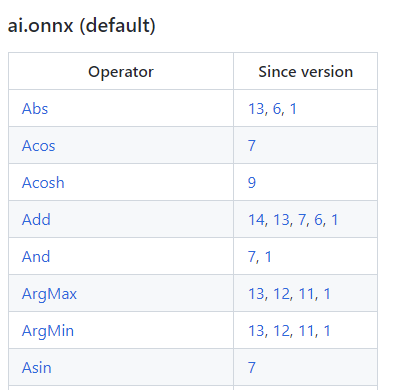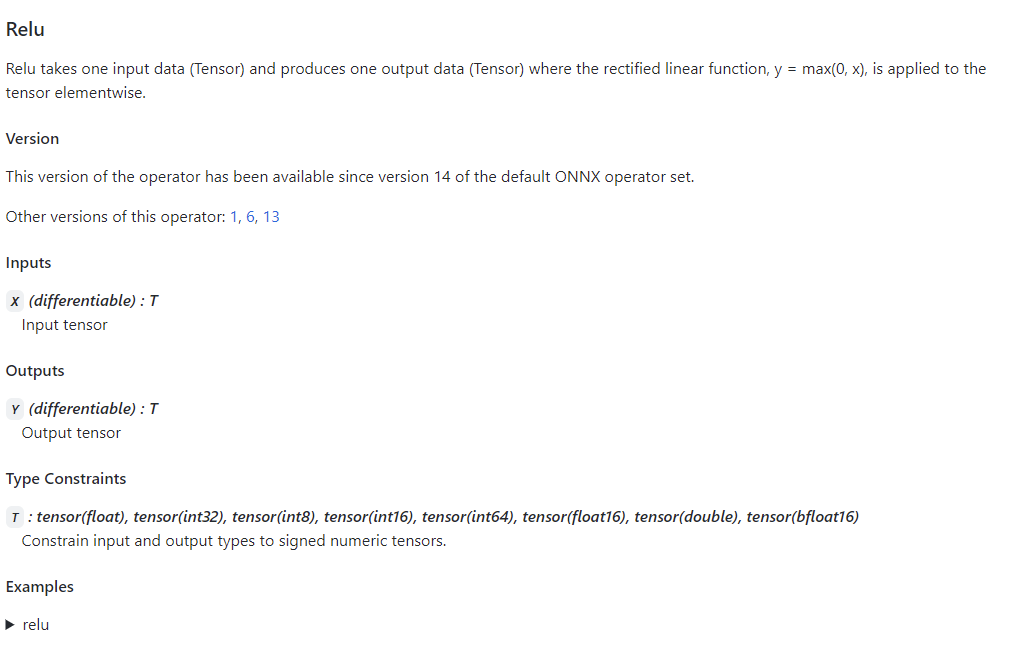### PyTorch 对 ONNX 算子的映射¶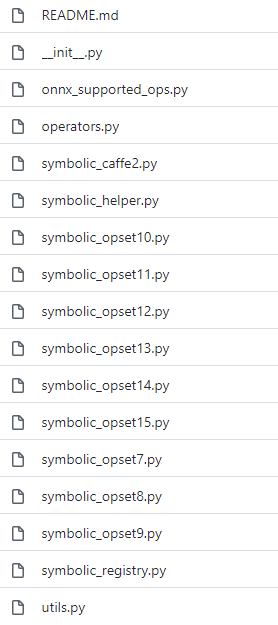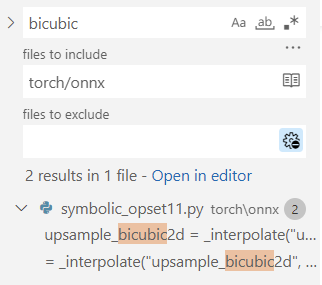upsample_bicubic2d = _interpolate("upsample_bicubic2d", 4, "cubic")

->

def _interpolate(name, dim, interpolate_mode):
return sym_help._interpolate_helper(name, dim, interpolate_mode)

->

def _interpolate_helper(name, dim, interpolate_mode):
def symbolic_fn(g, input, output_size, *args):
...

return symbolic_fn


    return g.op("Resize",
input,
empty_roi,
empty_scales,
output_size,
coordinate_transformation_mode_s=coordinate_transformation_mode,
cubic_coeff_a_f=-0.75,  # only valid when mode="cubic"
mode_s=interpolate_mode,  # nearest, linear, or cubic
nearest_mode_s="floor")  # only valid when mode="nearest"


## 总结¶

• 跟踪法和脚本化在导出带控制语句的计算图时有什么区别。

• torch.onnx.export()中该如何设置 input_names, output_names, dynamic_axes

• 使用 torch.onnx.is_in_onnx_export()来使模型在转换到 ONNX 时有不同的行为。

• 如何查询 ONNX 算子文档

• 如何查询 PyTorch 对某个 ONNX 版本的新特性支持情况。

• 如何判断 PyTorch 对某个 ONNX 算子是否支持，支持的方法是怎样的。

## 练习¶

1. Asinh 算子出现于第 9 个 ONNX 算子集。PyTorch 在 9 号版本的符号表文件中是怎样支持这个算子的？

2. BitShift 算子出现于第11个 ONNX 算子集。PyTorch 在 11 号版本的符号表文件中是怎样支持这个算子的？

3. 第一篇教程 中，我们讲过 PyTorch （截至第 11 号算子集）不支持在插值中设置动态的放缩系数。这个系数对应 torch.onnx.symbolic_helper._interpolate_helper的symbolic_fn的Resize算子映射关系中的哪个参数？我们是如何修改这一参数的？

© Copyright 2021-2024, OpenMMLab. Revision 1132e827.

Built with Sphinx using a theme provided by Read the Docs.
Versions
latest
stable
v1.2.0
v1.1.0
v1.0.0
0.x
v0.14.0